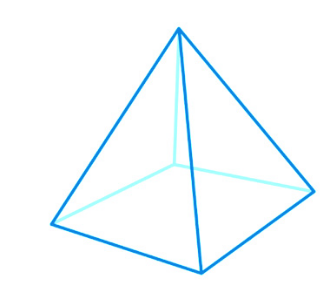# Regular Square Pyramid Formula

A regular square pyramid has a square base, 4 triangular faces, 5 vertices and 8 edges. Volume and Surface area are the major formulas for square pyramid. Let us discuss here both.The two major formulas for regular square pyramid are:

$\large Volume=\frac{1}{3}\, a^{2}h$

$\large Surface\;Area=a(a + \sqrt{a^{2}+4h^{2}})$

### Solved example

Question: Find the volume of a regular square pyramid of base length 6 cm and height 8 cm?

Solution:

Given, base length = a = 6 cm

Height = h = 8 cm

From the volume formula:

$$\begin{array}{l}Volume=\frac{1}{3}\, a^{2}h\end{array}$$

$$\begin{array}{l}Volume=\frac{1}{3}\times 6^{2}\times 8\end{array}$$

$$\begin{array}{l}Volume = 96\; cm^{3}\end{array}$$